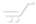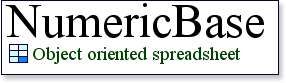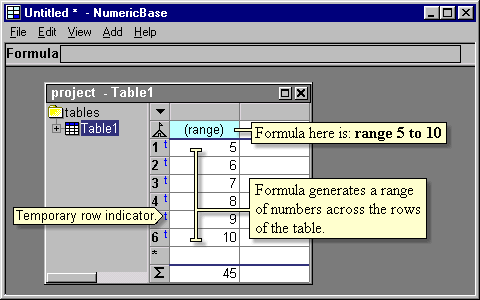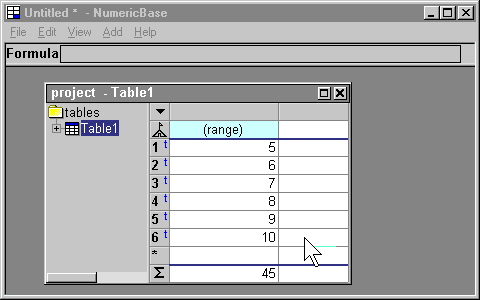# Range formula

Range formula
Range formulas let you generate a range of numbers using just one formula. The formula is placed at the base row and generate values across the rows of the table.Screenshot: range formula.

Temporary rows.
Some range formulas (called strong range formulas) require a minimum number of rows to display the range. If the table doesn't have this minimum number, then NumericBase adds temporary rows so the full range can be displayed. These temporary rows are labeled using the "temporary row indicator" as shown in the last screenshot (the indicator looks like this:).

The following animated screenshot shows how the temporary rows are automatically added as a response to an entry of a range formula.Animated screenshot: range formula adds temporary rows.

If the temporary rows are no longer needed, then they are removed automatically. For example, if we delete the range formula of the last animated screenshot, then the temporary rows will disappear.Animated screenshot: the temporary rows disappear.